March 21, 2019, at Baylor University

## Our team

• Dr. Chiung-Yu Huang
• Department of Epidemiology and Biostatistics
• University of California, San Francisco
• Dr. Kieren Marr
• School of Medicine
• Johns Hopkins University
• Dr. Gongjun Xu
• Department of Statistics
• University of Michigan, Ann Arbor
• Dr. Jun Yan
• Department of Statistics
• University of Connecticut

## Outline

• Motivation and background
• Existing methods
• Proposed model
• Estimation procedure
• Inference
• Simulation
• Transplant infections data
• Conclusion
• Reference

## Motivating data

• Transplant infections data
• Infections are common recurrent events that can contribute to death after transplant.
• Initiated in 2012 at the Johns Hopkins Hospital:
• Institutional Review Boards approved protocol.
• 161 kidney transplant recipients.
• Median follow-up time is 20.2 months.
• An average of 1.8 infection episodes per patient.
• Primary objective: Evaluate morbidity and mortality after transplant.

## Transplant infections data

• $$t_{ij}$$: the $$j$$th infection of the $$i$$th patient.
• $$Y_i$$: the terminal event time of the $$i$$th patient.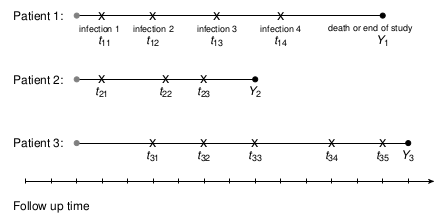• Patients were contacted roughly every 3 months.
• Patients were followed until death or the end of study.
• More infection $$\rightarrow$$ shorter survival time?

## Transplant infections data

• Of the 161 patients who received kidney transplant
• Age at transplant (categorized into ≥ 65 vs < 65 years).
• 31 were 65 years old or older
• Race (White vs other).
• 91 were white.
• Human leukocyte antigen (HLA)
• 31 received transplantation from a HLA incompatible donor.

## Visualization - Event plot

• reReg: rcurrent events regression .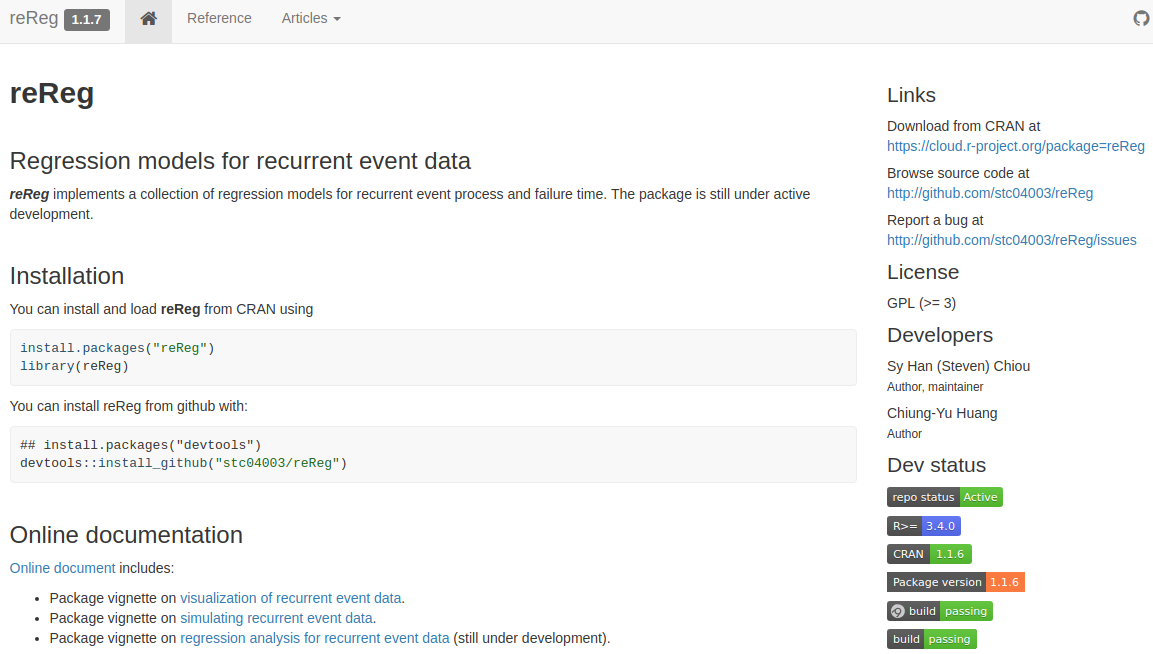## Event plot with reReg

• A snapshot of our data:
> dat
# A tibble: 246 x 7
id  Time event status   age race  HLA
<dbl> <dbl> <dbl>  <dbl> <dbl> <chr> <chr>
1     1   718     0      1     1 white HLA Compatible
2     2   717     0      1     0 white HLA Incompatible
3     3    29     1      0     0 white HLA Incompatible
4     3    85     1      0     0 white HLA Incompatible
5     3   106     1      0     0 white HLA Incompatible
6     3   155     1      0     0 white HLA Incompatible
7     3   204     1      0     0 white HLA Incompatible
8     3   227     1      0     0 white HLA Incompatible
9     3   318     1      0     0 white HLA Incompatible
10     3   468     0      1     0 white HLA Incompatible
# ... with 236 more rows

## Event plot with reReg

> plotEvents(reSurv(Time, id, event, status) ~ race, data = dat) +
+       theme(legend.position="none")
> plotEvents(reSurv(Time, id, event, status) ~ HLA, data = dat) +
+       theme(legend.position="none")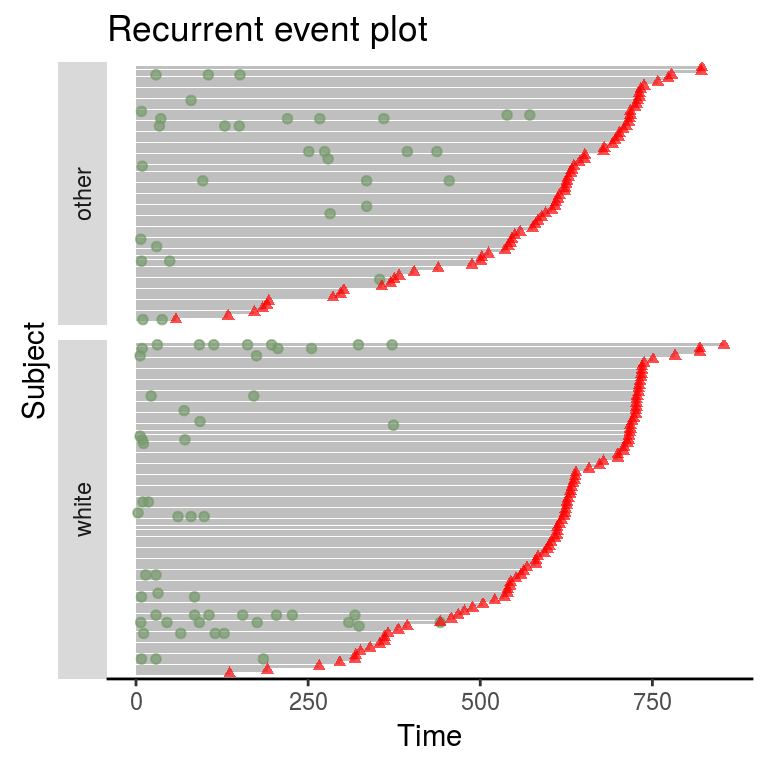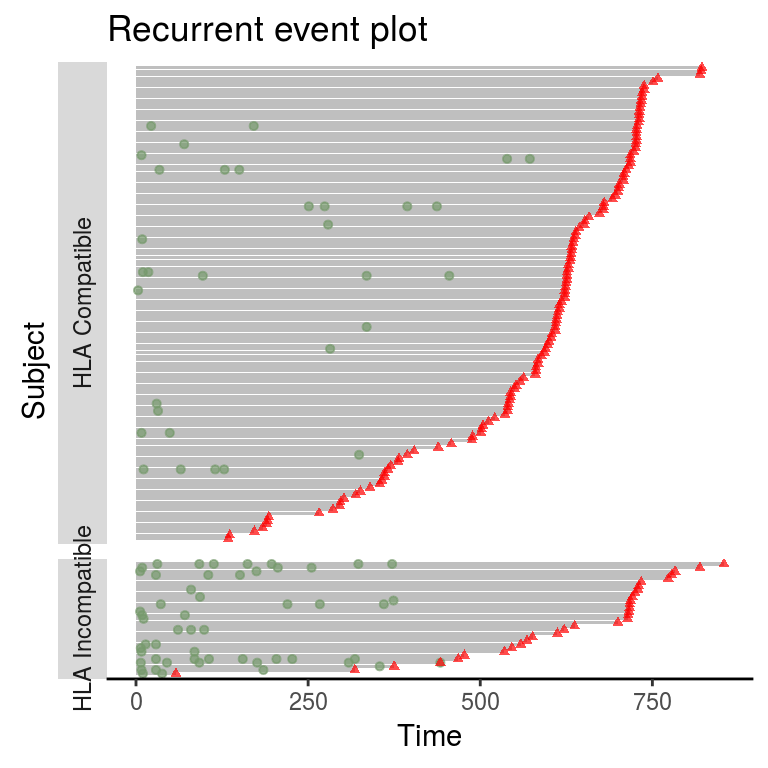## Notation

• $$N_i(t)$$: counting process of recurrent events of interest for the $$i$$th subject, $$i = 1, \ldots,n$$.
• $$t_{ij}$$: the $$j$$th infection of the $$i$$th patient.
• $$C_i$$: noninformative censoring time of the $$i$$th patient.
• $$D_i$$: informative censoring time of the $$i$$th patient.
• $$\tau$$: recurrent events can potentially be observed up to time $$\tau$$.
• $$Y_i$$: terminal event time of the $$i$$th patient, $$Y_i = \min(C_i, D_i, \tau)$$.
• $$m_i$$: number of events observed before time $$Y_i$$.
• $$X_i$$: the $$p\times1$$ covariate vector.

• Observed data are independent and identically distributed copies of $$\{N_i(t), Y_i, X_i: t\le Y_i, i = 1, \ldots, n\}.$$

## Existing methods for recurrent events

• Various regression models formulates the rate function for the counting process, given covariate: $$\lambda_i(t)d t = E\left[d N_i(t)| X_i\right]$$.
• Cox-type proportional rate models: $\lambda_i(t) = \lambda_0(t) e^{X_i^\top\beta}.$
• Pepe and Cai (1993),Lawless and Nadeau (1995), D. Lin et al. (2000).
• Covariates have a multiplicative effect on the baseline rate function.
• Accelerated rate model: $\lambda_i(t) = \lambda_0(t e^{X_i^\top\beta}).$
• Chen and Wang (2000) and Ghosh (2004).
• Covariate modify the time-scale on the baseline rate function.

## Existing methods for recurrent events

• Accelerated mean model: $\lambda_i(t) = \lambda_0(t e^{X_i^\top\beta}) e^{X_i^\top\beta}.$
• D. Y. Lin, Wei, and Ying (1998)
• $$E\{N_i(t)|X_i\}=\Lambda_0(te^{X_i^\top\alpha})$$.
• Covariates modify the time scale of the cumulative mean function.
• General class model: $\lambda_i(t) = \lambda_0(t e^{X_i^\top\alpha}) e^{X_i^\top\beta}.$
• Sun and Su (2008)
• Two types of covariate effects:
• Scale-change effect that alters the time scale.
• Multiplicative effect.

## Existing methods for recurrent events

• These methods require a noninformative censoring assumption
• Failing to account for informative censoring $$\rightarrow$$ substantial bias in inferences
• Cook and Lawless (1997),Ghosh and Lin (2002),Luo, Wang, and Huang (2010).
• Joint frailty models:
• Ye, Kalbfleisch, and Schaubel (2007),Zeng and Lin (2009), etc.
• Captures the association between $$Y_i$$ and $$N_i(t)$$.
• Accounts for heterogeneity that cannot be explained by $$X_i$$.
• Often requires a parametric assumption on the frailty distribution.
• Performance depends on correct modeling of the terminal event.
> args(reReg)
function (formula, data, B = 200, method = c("cox.LWYY", "cox.GL",
"cox.HW", "am.GL", "am.XCHWY", "sc.XCYH"), se = c("NULL",
"bootstrap", "resampling"), contrasts = NULL, control = list())
NULL

## Proposed model

• We formulate the rate function for $$N_i(t)$$, given $$X_i$$ and an unobserved subject-specific nonnegative frailty $$Z_i$$: $$\lambda_i(t) d t= E[d N_i(t) \mid Z_i,X_i]$$, $\lambda_i(t) = Z_i\lambda_0(te^{X_i^\top\alpha})e^{X_i^\top\beta}, t\in[0, \tau].$

• We assume $$Y_i\perp N_i(\cdot)|(Z_i, X_i)$$, without specifying a model for $$Y_i$$.
• $$Z_i$$ has an unspecified distribution with $$E(Z_i^2)<\infty$$.
• $$Z_i$$ inflates (or deflates) the occurrence rate of recurrent events.
• Identifiability condition:
• $$\int_0^\tau\lambda_0(u)\,d u = 1$$.
• $$E(Z_i|X_i) = \mu_z$$ is an unknown constant.

## Proposed model

• Our model includes many existing models as special cases:
• $$\alpha = 0$$ Wang, Qin, and Chiang (2001), Huang and Wang (2004): $\lambda_i(t) =Z_i \lambda_0(t )e^{X_i^\top\beta}.$
• $$\beta = 0$$ Ghosh (2004): $\lambda_i(t) =Z_i \lambda_0(t e^{X_i^\top\alpha }).$
• $$\alpha = \beta$$ Xu et al. (2017): $\lambda_i(t) =Z_i \lambda_0(te^{X_i^\top\alpha} )e^{ X_i^\top\alpha}.$
• Our model also allows model selection through testing $$H_o: \alpha = 0$$, $$H_o: \beta = 0$$, and $$H_o: \alpha = \beta$$.

## Estimation procedure

• Two step estimation: $$\alpha$$ and $$\Lambda_0(t) = \int_0^t\lambda_0(u)d u$$, then $$\beta$$.
• $$Z_i$$ is an unobserved frailty that does not need to be estimated.
• Does not require a Poisson assumption.
• Does not need to model terminal events.

## Estimation of $$\alpha$$ and $$\Lambda_0$$

• Consider the transformation $$t^*_{ij} = t_{ij}e^{X_i^\top \alpha}$$, $$Y_i^* = Y_ie^{X_i^\top \alpha}$$
• The counting process on the transformed time scale. $N_i^*(t) = \sum_{j = 1}^{m_i} I(t_{ij}^*\le t) = \sum_{j = 1}^{m_i}I(t_{ij}\le te^{X_i^\top \alpha}) = N_i(te^{X_i^\top \alpha}).$
• This implies $\begin{equation} E\{N_i^*(t)|X_i, Z_i, Y_i^*\} = Z_i\Lambda_0(t)e^{X_i^\top (\beta-\alpha)}, \,\,\,\,\,\,(1) \end{equation}$ the mean function follows the Cox-type proportional model with a multiplicative frailty.

## Estimation of $$\alpha$$ and $$\Lambda_0$$

• Condition on $$\{X_i, Z_i, Y_i, m_i\}$$, the $$m_i$$ event times $$t^*_{ij}$$'s can be seem as order statistics of iid random variables with (truncation) density function: $\frac{Z_i\lambda_0(t)e^{-X_i^\top (\beta-\alpha)}}{Z_i\Lambda_0(Y_i^*)e^{-X_i^\top (\beta-\alpha)}} =\frac{\lambda_0(t)}{\Lambda_0(Y^*_i)}, t \le Y_i^*.$
• This technique was first applied by Wang, Qin, and Chiang (2001).
• This (truncation) density implies $E\{N_i^*(t)|X_i, Z_i, Y_i^*, m_i\} = \sum_{j=1}^{m_i}P(t_{ij}^*\le t) = m_i\frac{\Lambda_0(t)}{\Lambda_0(Y_i^*)}, t\le Y_i^\ast.\,\, (2)$
• $$E\{m_i(t)|X_i, Z_i, Y_i^*\} = Z_i\Lambda(Y_i^*)e^{X_i^\top(\beta-\alpha)}$$, (2) implies (1).
• This motivates us to extend methods for right-truncated survival data Wang (1989) to construct estimating equation.

## Estimation of $$\alpha$$ and $$\Lambda_0$$

• We derive a zero mean stochastic process $M_i^*(t) = N_i^*(t) - \int_0^tR_i^*(u)d H(u),$ for $$R_i^*(t) = \sum_{j=1}^{m_i}I\{t_{ij}^*\le t\le Y_i^*\}$$, $$H(t) = \log \Lambda_0(t)$$.

• $$M_i^*(t)$$ is a zero mean stochastic process, we have $$E\left\{ \sum_{i=1}^n\int_0^td M_i^*(u) \right\}=0$$ and $$E\left\{ \sum_{i=1}^n\int_0^tX_i\,d M_i^*(u) \right\}=0$$.

## Estimation of $$\alpha$$ and $$\Lambda_0$$

• This gives us two estimating equations: $n^{-1}\sum_{i=1}^n\int_0^\infty\left\{X_i - \frac{\sum_{j = 1}^nX_jR_j^*(t)}{\sum_{j = 1}^nR_j^*(t)}\right\}d N_i^*(t) = 0$ and $\widehat H_n(t, a) = -\int_t^\infty\frac{\sum_{i=1}^nd N_i^*(u)}{\sum_{i=1}^nR_i^*(u)}.$
• $$\Lambda_0(t)$$ can be estimated by $$\exp\{\widehat H_n(t)\}$$.

## Estimation of $$\beta$$

• Under our model $$\lambda_i(t) = Z_i\lambda_0(te^{X_i^\top\alpha})e^{X_i^\top\beta}$$, $$m_i$$ satisfies $E(m_i|X_i, Y_i^*, Z_i) = Z_i\Lambda_0(Y_i^*)e^{X_i^\top(\beta-\alpha)}$
• This gives an estimating equation to solve for $$\beta$$: $n^{-1}\sum_{i=1}^nX_i\left[m_i\hat\Lambda_n^{-1}\{Y_i^*(\hat \alpha_n)\} - e^{X_i^\top(\beta - \hat\alpha_n)}\right]=0$

## Inference

• We use perturbed estimating functions to estimate the target sandwich variance estimator: $$\sum = J_{\alpha, \beta}^{-1}V_{\alpha, \beta}J_{\alpha, \beta}^{-1}$$.
> args(reReg)
function (formula, data, B = 200, method = c("cox.LWYY", "cox.GL",
"cox.HW", "am.GL", "am.XCHWY", "sc.XCYH"), se = c("NULL",
"bootstrap", "resampling"), contrasts = NULL, control = list())
NULL
• $$n^{1/2}(\hat \alpha_n - \alpha)$$ and $$n^{1/2}(\hat \beta_n - \beta)$$ converge weakly to a zero mean multivariate normal distribution.
• $$n^{1/2}\{\hat\Lambda_n(t, \hat\alpha_n) - \Lambda_0(t)\}$$, $$t\in[0,\tau]$$, converges weakly to a mean-zero Gaussian process.

## Simulation 1

• Recurrent event process from a non-stationary Poisson process with rate $\lambda(t) = Z\lambda_0(te^{\alpha_1X_1 + \alpha_2X_2})e^{\beta_1X_1 + \beta_2X_2}.$
• Baseline rate function $$\lambda_0(t) = [2(1+t)]^{-1}$$.
• $$X_1$$ and $$X_2$$ were generated from independent standard normal.
• $$Y_i=\min(60e^{-X_1}/Z, 60)$$.
• Scenarios:
• $$Z = 1$$ or $$Z\sim Gamma(4, 4)$$.
• $$n = 200, 400.$$
• $$\alpha_1 = \alpha_2 = -1$$, $$\beta_1 = \beta_2=1$$.
• Average observed recurrent event ranges from 1.5 to 5.7.
• Compare results to Sun and Su (2008), which requires a noninformative censoring assumption.
• 1000 replicates.

## Simulation 1

> args(simSC)
function (n, a, b, indCen = TRUE, type = c("cox", "am", "sc"),
tau = 60, zVar = 0.25, summary = FALSE)
NULL
> invisible(simSC(200, c(-1, -1), c(1, 1), summary = TRUE))

Summary results for number of recurrent event per subject:
Min. 1st Qu.  Median    Mean 3rd Qu.    Max.
0.00    1.00    3.00    6.81    8.00   99.00

Number of failures: 91 (45.5%); Number of censored events: 109 (54.5%)

Number of x1 == 1: 99 (49.5%); Number of x1 == 0: 101 (50.5%)
Summary results for x2:
Min. 1st Qu.  Median    Mean 3rd Qu.    Max.
-2.7018 -0.7563 -0.1014 -0.1015  0.6459  2.0143 

## Simulation 1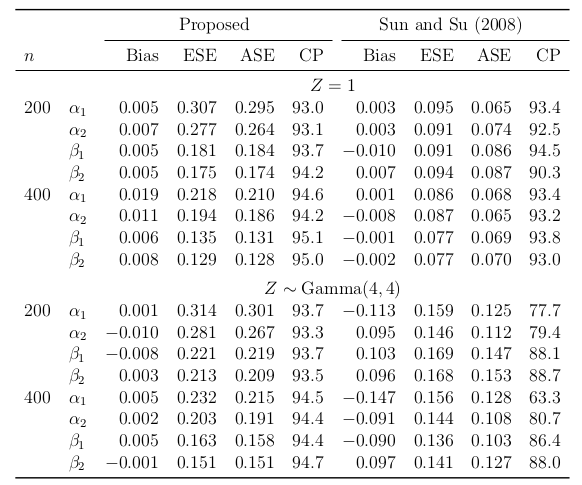## Simulation 2

• Investigate the performance of the hypothesis.
• Two scenarios:
• Hold $$\alpha_1 = \alpha_2 = 0$$, vary $$\beta_1 = \beta_2 = K$$, where $$K\in[-1,1]$$.
• Hold $$\beta_1 = \beta_2 = 0$$, vary $$\alpha_1 = \alpha_2 = K$$, where $$K\in[-1,1]$$.
• For each configuration of $$(\alpha_1, \alpha_2)$$ and $$(\beta_1, \beta_2)$$, we test
• $$H_o:\alpha = 0$$; Cox-type model {$$\lambda(t) = Z\lambda_0(t) e^{X^\top\beta}$$.}
• $$H_o: \beta=0$$; Accelerated rate model {$$\lambda(t) = Z\lambda_0(te^{X^\top\alpha})$$.}
• $$H_o:\beta - \alpha = 0$$; Accelerated mean model $$\lambda(t) = Z\lambda_0(te^{X^\top\beta})e^{X^\top\beta}$$.
• Compute empirical power based on 1000 replicates.

## Simulation 2

• Rejection rates based on 1000 replications at 0.05 significance level.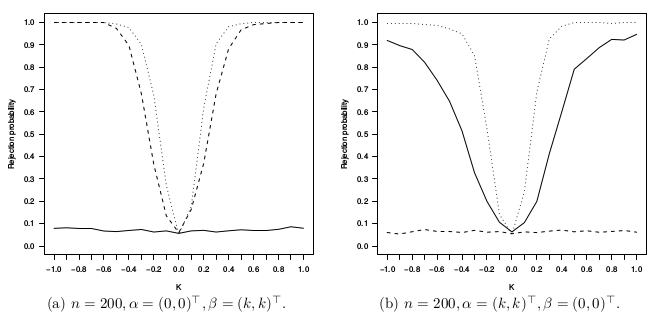• Solid lines: $$H_0: \alpha = 0$$ (Cox assumption).
• Dashed lines: $$H_0: \beta = 0$$ (accelerated rate assumption).
• Dotted lines: $$H_0: \gamma = 0$$ (accelerated mean assumption).

## Transplant infections data

> fit <- reReg(reSurv(Time, id, event, status) ~ age + race + HLA,
>              data = dat, method = "sc.XCYH")
> summary(fit)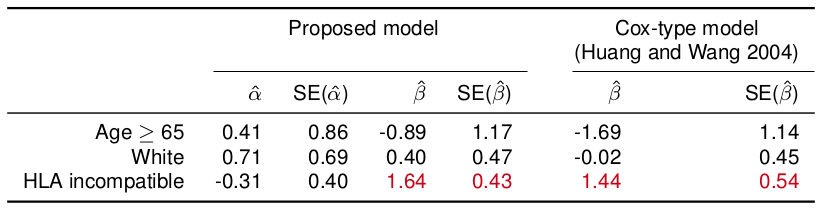• $$H_o: \alpha = 0$$ gives a $$p$$-value of 0.52.
• $$H_o: \beta = 0$$ and $$H_o: \beta - \alpha = 0$$ are rejected at $$\alpha = 0.01$$.
• Patients with HLA incompatibility is expected to have 4.22 times more infections.

## Future works

• Multivariate recurrent events
• Patients experienced different types of infections.
• Bloodstream infections, viral infections, gastrointestinal infection.
• Application to panel count
• Skin cancer chemoprevention trail conducted at the University of Wisconsin Comprehensive Cancer Center Bailey et al. (2010).
• The occurrence of skin tumors is the recurrent event.
• Only the numbers of events that occur between successive examination times are observed.

## Reference

Bailey, Howard H, KyungMann Kim, Ajit K Verma, Karen Sielaff, Paul O Larson, Stephen Snow, Theresa Lenaghan, Jaye L Viner, Jeff Douglas, and Nancy E Dreckschmidt. 2010. “A Randomized, Double-Blind, Placebo-Controlled Phase 3 Skin Cancer Prevention Study of $$\alpha$$-Difluoromethylornithine in Subjects with Previous History of Skin Cancer.” Cancer Prevention Research 3 (1). AACR: 35–47.

Chen, Ying Qing, and Mei-Cheng Wang. 2000. “Estimating a Treatment Effect with the Accelerated Hazards Models.” Controlled Clinical Trials 21 (4). Elsevier: 369–80.

Cook, Richard J, and Jerald F Lawless. 1997. “Marginal Analysis of Recurrent Events and a Terminating Event.” Statistics in Medicine 16 (8). Wiley Online Library: 911–24.

Ghosh, Debashis. 2004. “Accelerated Rates Regression Models for Recurrent Failure Time Data.” Lifetime Data Analysis 10 (3): 247–61.

Ghosh, Debashis, and DY Lin. 2002. “Marginal Regression Models for Recurrent and Terminal Events.” Statistica Sinica 12 (3): 663–88.

Huang, Chiung-Yu, and Mei-Cheng Wang. 2004. “Joint Modeling and Estimation for Recurrent Event Processes and Failure Time Data.” Journal of the American Statistical Association 99 (468): 1153–65.

Lawless, Jerald F, and Claude Nadeau. 1995. “Some Simple Robust Methods for the Analysis of Recurrent Events.” Technometrics 37 (2). Taylor & Francis: 158–68.

Lin, D Y, L J Wei, and Zhiliang Ying. 1998. “Accelerated Failure Time Models for Counting Processes.” Biometrika 85 (3): 605–18.

Lin, DY, LJ Wei, I Yang, and Z Ying. 2000. “Semiparametric Regression for the Mean and Rate Functions of Recurrent Events.” Journal of the Royal Statistical Society: Series B 62 (4). Wiley Online Library: 711–30.

Luo, Xianghua, Mei-Cheng Wang, and Chiung-Yu Huang. 2010. “A Comparison of Various Rate Functions of a Recurrent Event Process in the Presence of a Terminal Event.” Statistical Methods in Medical Research 19 (2): 167–82.

Pepe, Margaret Sullivan, and Jianwen Cai. 1993. “Some Graphical Displays and Marginal Regression Analyses for Recurrent Failure Times and Time Dependent Covariates.” Journal of the American Statistical Association 88 (423). Taylor & Francis: 811–20.

Sun, Liuquan, and Bin Su. 2008. “A Class of Accelerated Means Regression Models for Recurrent Event Data.” Lifetime Data Analysis 14 (3): 357–75.

Wang, Mei-Cheng. 1989. “A Semiparametric Model for Randomly Truncated Data.” Journal of the American Statistical Association 84 (407). Taylor & Francis: 742–48.

Wang, Mei-Cheng, Jing Qin, and Chin-Tsang Chiang. 2001. “Analyzing Recurrent Event Data with Informative Censoring.” Journal of the American Statistical Association 96 (455): 1057–65.

Xu, Gongjun, Sy Han Chiou, Chiung-Yu Huang, Mei-Cheng Wang, and Jun Yan. 2017. “Joint Scale-Change Models for Recurrent Events and Failure Time.” Journal of the American Statistical Association. Taylor & Francis.

Ye, Yining, John D Kalbfleisch, and Douglas E Schaubel. 2007. “Semiparametric Analysis of Correlated Recurrent and Terminal Events.” Biometrics 63 (1): 78–87.

Zeng, Donglin, and DY Lin. 2009. “Semiparametric Transformation Models with Random Effects for Joint Analysis of Recurrent and Terminal Events.” Biometrics 65 (3): 746–52.